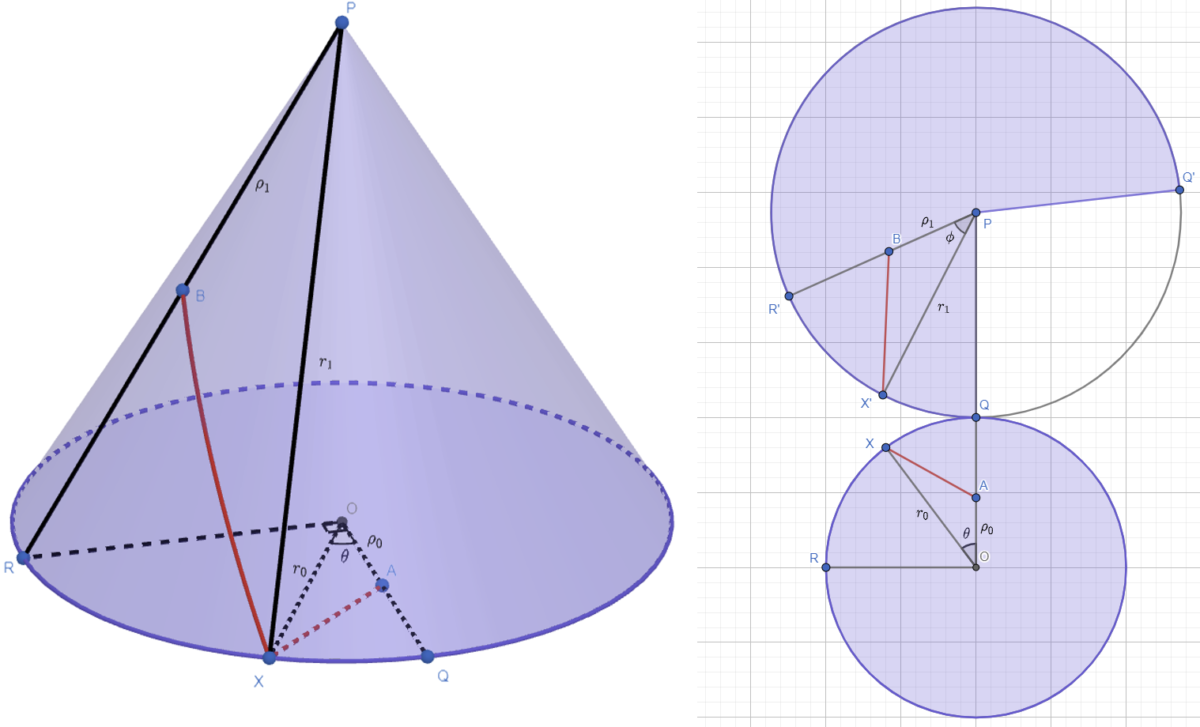# Cone crawlingThis week’s Riddler Classic is a geometry problem about traversing the surface of a cone

The circular base of the cone has a radius of 2 meters and a slant height of 4 meters. We start on the base, a distance of 1 meter away from the center. The goal is to reach the point half-way up the cone, 90 degrees around the cone’s central axis from the start, as shown. What is the shortest path?

Here is my solution:
[Show Solution]

## 3 thoughts on “Cone crawling”

1.Guy Moore says:

Alternatively, the angle OXA and the angle PX’B must be equal — because the curve should also be straight at the point where it crosses from the base to the cone. (This is sort of like Snell’s Law.)
That eliminates having to differentiate to optimize the two angles….

1.Laurent says:

Good point! I updated my post to include the simpler equations and the equal-angle intuition. I actually thought of Snell’s law when I was writing up my solution, but I didn’t connect all the dots at the time. This does lead to simpler equations, but I still don’t see a general analytic solution. Seems that’s only possible in special cases, e.g. as in the problem statement when the various radii are rational multiples of one another.

2.B Fortner says:

omg, I forgot about Snell’s Law / Fermat’s Principle